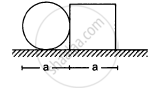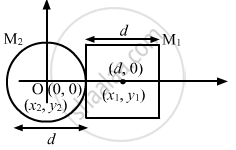Advertisement Remove all ads

# A Square Plate of Edge D and a Circular Disc of Diameter D Are Placed Touching Each Other at the Midpoint of an Edge of the Plate as Shown in Figure. - Physics

Sum

A square plate of edge d and a circular disc of diameter d are placed touching each other at the midpoint of an edge of the plate as shown in figure. Locate the centre of mass of the combination, assuming same mass per unit area for the two plates.Advertisement Remove all ads

#### Solution

Let m be the mass per unit area of the square plate and the circular disc .$\Rightarrow$ Mass of the square plate, M1 = d2m
Mass of the circular disc, M2 = $\frac{\pi d^2}{4}m$
Let the centre of the circular disc be the origin of the system.
$\Rightarrow$   x1 = d, y1 = 0
x2 = 0, y2 = 0
$\Rightarrow$ Position of the centre of mass of circular disc and square plate:
$= \left( \frac{m_1 x_1 + m_2 x_2}{m_1 + m_2}, \frac{m_1 y_1 + m_2 y_2}{m_1 + m_2} \right)$
$= \left( \frac{d^2 md + \pi\left( d^2 /4 \right) m \times 0}{d^2 m + \pi \left( d^2 /4 \right) m}, \frac{0 + 0}{m_1 + m_2} \right)$
$= \left( \frac{d^3 m}{d^2 m(1 + \pi/4)}, 0 \right)$
$= \left( \frac{4d}{\pi + 4}, 0 \right)$
Hence, the new centre of mass of the system (circular disc plus square plate) lies at distance $\frac{4d}{(\pi + 4)}$  from the centre of circular disc, towards right.

Is there an error in this question or solution?
Advertisement Remove all ads

#### APPEARS IN

HC Verma Class 11, 12 Concepts of Physics 1
Chapter 9 Centre of Mass, Linear Momentum, Collision
Q 6 | Page 160
Advertisement Remove all ads
Advertisement Remove all ads
Share
Notifications

View all notifications

Forgot password?